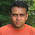## Introduction

Dataset and datatable are the lifelines of asp.net (c#). Without data table & dataset we are short, inflexible, expensive, unmanageable and less availability. Recently I dealt greatly with transposed datatable in C#. Data was throwing me in vertical and I need to manipulate data back into horizontally. This is very time consuming to manage the data properly according to our situation, so it needs me to manage transpose datatable in c# according to situation; moreover I motivated to share this one in detail. I have provided steps how can we generate transpose datatable in c# as below:
`using System.Data;`
Step 1: In first step, I have added some required .net controls to view transpose data table in Gridview.

Step 2: I have added some dummy data into inputTable:

```protected void tc_button_Click(object sender, EventArgs e)
{
DataTable inputTable = new DataTable();

inputTable.TableName = "InputTable";

DataTable transposedTable = GenerateTransposedTableinCsharp(inputTable);
transposedTable.TableName = "TC-TransponsedTable";
transposedTable.Columns.Remove("Name");
tc_gvresults.DataSource = transposedTable;
tc_gvresults.Visible = true;
tc_gvresults.DataBind();
}
```

Step 3: Here I have written complete method GenerateTransposedTableinCsharp to generate transpose datatable in C#.
```private DataTable GenerateTransposedTableinCsharp(DataTable inputTable)
{
DataTable outputTable = new DataTable();

foreach (DataRow inRow in inputTable.Rows)
{
string newColName = inRow.ToString();
DataColumnCollection columns = outputTable.Columns;

if (columns.Contains(newColName))
{
// do nothing
}
else
{
}
}

Int32 iColCount = Convert.ToInt32(iTotalRows(inputTable));
string _Col = string.Empty;
string _ColRep = string.Empty;
Int32 _rCount = 0;
Int32 _FirstRow = 0;
Int32 _NewRow = 0;

for (int rCount = 0; rCount <= iColCount; rCount++)
{
DataRow newRow = outputTable.NewRow();

newRow = inputTable.Columns.ColumnName.ToString();
for (int cCount = _rCount; _rCount <= inputTable.Rows.Count - 1; cCount++)
{
if (_FirstRow == 0)
{
_ColRep = inputTable.Rows[cCount].ToString();
}

if (_FirstRow > 0)
{
_Col = inputTable.Rows[cCount].ToString();
}
_FirstRow += 1;

if (_ColRep == _Col)
{
_rCount = cCount;
_FirstRow = 0;
_NewRow = 0;
_Col = "";
break;
}
else
{
string colValue = inputTable.Rows[cCount].ToString();
newRow[_NewRow + 1] = colValue;
}
_rCount = _rCount + 1;
_NewRow = _NewRow + 1;
}
}
return outputTable;
}
}
```

### I have written under above code a helper method to write rows to columns:

```  private decimal iTotalRows(DataTable inputTable)
{
int _FirstRow = 0;
string _ColRep = "";
string _Col = "";
Decimal _RetRows = 0;
Int32 _TotalRecs = Convert.ToInt32(inputTable.Rows.Count - 1);

for (int cCount = 0; cCount <= inputTable.Rows.Count - 1; cCount++)
{
if (_FirstRow == 0)
{
_ColRep = inputTable.Rows[cCount].ToString();
}

if (_FirstRow > 0)
{
_Col = inputTable.Rows[cCount].ToString();
}
if (_ColRep == _Col)
{
Int32 iTotRow = cCount;
iTotRow = iTotRow + 1;
//string _RRows;
_RetRows = Convert.ToDecimal(Convert.ToDecimal(_TotalRecs) / Convert.ToDecimal(iTotRow));
_RetRows = Math.Ceiling(_RetRows);
break;
}
_FirstRow += 1;
}

return _RetRows;
}
```

Step 4: Now, I have written completely code in code behind and HTML. Next I am ready to hit button “Hit Me!” to bind results into GridView control in C#.

Step 5: After hitting the button, now transpose table perfectly showing in gridview.

Above key steps show how can we generate transpose datatable in c#, if any help more required on it, I am very happy to assist my digital world.Share### Anjan Kant

Outstanding journey in Microsoft Technologies (ASP.Net, C#, SQL Programming, WPF, Silverlight, WCF etc.), client side technologies AngularJS, KnockoutJS, Javascript, Ajax Calls, Json and Hybrid apps etc. I love to devote free time in writing, blogging, social networking and adventurous life

1.1.